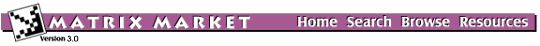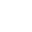## LAPACK Test Matrix Generators DLATMR/ZLATMR: A CGI Interface

### from set XLATMR

[Description] [Interactive Matrix/Driver Generation]

### DLATMR/ZLATMR Description:

(from LAPACK documentation)

DLATMR/ZLATMR generates random matrices of various types for testing LAPACK programs by applying the following sequence of operations:
1. Generate a matrix A with random entries of distribution DIST which is symmetric if SYM='S', and nonsymmetric if SYM='N'.
2. Set the diagonal to D, where D may be input or computed according to MODE, COND, DMAX and RSIGN as described below.
3. Grade the matrix, if desired, from the left and/or right as specified by GRADE. The inputs DL, MODEL, CONDL, DR, MODER and CONDR also determine the grading as described below.
4. Permute, if desired, the rows and/or columns as specified by PIVTNG and IPIVOT.
5. Set random entries to zero, if desired, to get a random sparse matrix as specified by SPARSE.
6. Make A a band matrix, if desired, by zeroing out the matrix outside a band of lower bandwidth KL and upper bandwidth KU.
7. Scale A, if desired, to have maximum entry ANORM.
8. Pack the matrix if desired. Options specified by PACK are:
• no packing
• store the lower half columnwise (if symmetric or Hermitian)

### Input for Matrix/Driver generation:

The following form allows you to generate a matrix using the LAPACK routine DLATMR/ZLATMR. Make any changes to default parameters, and then press the Generate Matrix button. The matrix, in Matrix Market format, will be returned to your browser. See documentation within dlatmr.f and zlatmr.f for a complete description of all available parameters.

Alternately, you may generate a sample Fortran driver based on your input by pressing the Generate Sample Driver button.

• The components from the LAPACK TESTING distribution necessary for building a dlatmr/zlatmr driver are available here as Gzipped, shar-ed source code.
• To assist in compiling the generated sample driver programs, a sample makefile is available.

### DLATMR/ZLATMR Calling Sequence:SUBROUTINE xLATMR ( M, N, DIST, ISEED, SYM, D, MODE, COND, DMAX, RSIGN, GRADE, DL, MODEL, CONDL, DR, MODER, CONDR, PIVTNG, IPIVOT, KL, KU, SPARSE, ANORM, PACK, A, LDA, IWORK, INFO )

FIELD'D' = double precision 'Z' = double precision complex
Parameters:
MThe number of rows of A: ( 1 <= M <= 250 )
NThe number of columns of A: ( 1 <= N <= 250 )
DISTType of distribution to be used to generate a random matrix:
'S' = Uniform (-1, 1) 'U' = Uniform ( 0, 1) 'N' = Normal ( 0, 1)
ISEEDCalculate random seed values
User specified seed values:
(If specifying, choose integers between 0 and 4095 inclusive with ISEED(4) odd.)
SYMSymmetry of generated matrix:
'S' = Symmetric 'H' = Hermitian 'N' = Nonsymmetric
If SYM is 'S' or 'H', storage may be reduced by half by only storing lower triangular entries. See parameter 'PACK'.
DDiagonal entries. (Disabled)
MODEDiagonal selection: (MODE = 0 (diagonal input) is disabled)
 (1) D(1)=1; D(2:N)=1.0/COND (-1) D(N)=1; D(1:N-1)=1.0/COND (2) D(1:N-1)=1; D(N)=1.0/COND (-2) D(1)=1.0/COND; D(2:N)=1 (3) D(I)=COND**(-(I-1)/(N-1)) (-3) D(I)=COND**(-(N-I)/(N-1)) (4) D(I)=1 - (I-1)/(N-1)*(1 - 1/COND) (-4) D(I)=1 - (N-I)/(N-1)*(1 - 1/COND) (5) ranging from 1/COND to 1 with uniformly distributed logarithms (-5) ranging from 1 to 1/COND with uniformly distributed logarithms (6) same distribution as the rest of the matrix (low to high) (-6) same distribution as the rest of the matrix (high to low)
CONDParameter for diagonal selection (above):
(if used, must be >= 1)
DMAXScaling factor for diagonal selection:
(for COMPLEX matrices, enter two values: real and imaginary parts)
If MODE is neither -6, 0 nor 6, the diagonal is scaled by DMAX / max(abs(D(I))), so that maximum absolute entry of diagonal is abs(DMAX). If DMAX is negative (or zero), diagonal will be scaled by a negative number (or zero).
RSIGNMultiply, or do NOT multiply diagonal by random sign
GRADE'N' = No grading
'L' = diag(DL) · A (for nonsymmetric matrices)
'R' = A · diag(DR) (for nonsymmetric matrices)
'B' = diag(DL) · A · diag(DR) (for nonsymmetric matrices)
'S' = diag(DL) · A · diag(DL) (for symmetric matrices)
'H' = diag(DL) · A · diag(DL) (for Hermitian matrices)
'E' = diag(DL) · A · inv(diag(DL)) (eigenvalue invariance, for nonsymmetric matrices, and M must equal N)
DLLeft (row) scaling entries. (Disabled)
MODELLeft grading matrix selection: (MODE = 0 (grading input) is disabled)
 (1) DL(1)=1; DL(2:N)=1.0/COND (-1) DL(N)=1; DL(1:N-1)=1.0/COND (2) DL(1:N-1)=1; DL(N)=1.0/COND (-2) DL(1)=1.0/COND; DL(2:N)=1 (3) DL(I)=COND**(-(I-1)/(N-1)) (-3) DL(I)=COND**(-(N-I)/(N-1)) (4) DL(I)=1 - (I-1)/(N-1)*(1 - 1/COND) (-4) DL(I)=1 - (N-I)/(N-1)*(1 - 1/COND) (5) ranging from 1/COND to 1 with uniformly distributed logarithms (-5) ranging from 1 to 1/COND with uniformly distributed logarithms (6) same distribution as the rest of the matrix (low to high) (-6) same distribution as the rest of the matrix (high to low)
CONDLParameter for left grading selection (above):
(if used, must be >= 1)
DRRight (column) scaling entries. (Disabled)
MODERRight grading matrix selection: (MODE = 0 (grading input) is disabled)
 (1) DR(1)=1; DR(2:N)=1.0/COND (-1) DR(N)=1; DR(1:N-1)=1.0/COND (2) DR(1:N-1)=1; DR(N)=1.0/COND (-2) DR(1)=1.0/COND; DR(2:N)=1 (3)DR(I)=COND**(-(I-1)/(N-1)) (-3)DR(I)=COND**(-(N-I)/(N-1)) (4) DR(I)=1 - (I-1)/(N-1)*(1 - 1/COND) (-4) DR(I)=1 - (N-I)/(N-1)*(1 - 1/COND) (5) ranging from 1/COND to 1 with uniformly distributed logarithms (-5) ranging from 1 to 1/COND with uniformly distributed logarithms (6) same distribution as the rest of the matrix (low to high) (-6) same distribution as the rest of the matrix (high to low)
CONDRParameter for right grading selection (above):
(if used, must be >= 1)
PIVTNGSpecify the pivoting permutations. (Disabled)
IPIVOTPivot vector entries. (Disabled)
KLLower bandwidth:
For example, KL=0 implies upper triangular, KL=1 implies upper Hessenberg, and KL at least M-1 implies the matrix is not banded. Must equal KU if matrix is symmetric. If left blank, M-1 will be assumed (unless overridden by specified KL with symmetry).
KUUpper bandwidth:
For example, KU=0 implies lower triangular, KU=1 implies lower Hessenberg, and KU at least N-1 implies the matrix is not banded. Must equal KL if matrix is symmetric. If left blank, N-1 will be assumed (unless overridden by specified KU with symmetry).
SPARSEThe degree of sparsity of the matrix (from 0.0 to 1.0, where 0.0 implies dense):
(0.0 will be assumed if left blank).
This interface allows for data to be returned in Matrix Market coordinate format. Check here to select coordinate storage (recommended when SPARSE > .6).
ANORMScale output matrix so that the maximum entry is:
Do not scale output matrix
PACKMatrix packing: (several packing options disabled)
'R' = Symmetric storage (lower triangle) 'N' = No packing
AReturned matrix data. (Disabled)
LDALeading dimension of A. (Disabled)
IWORKInteger workspace of dimension M or N. (Disabled)
INFOReturn code. (Disabled)

[ Home ] [ Search ] [ Browse ] [ Resources ] [ XLATMR ]

Last change in this page: Mon Apr 14 18:24:02 US/Eastern 1997 [ ]